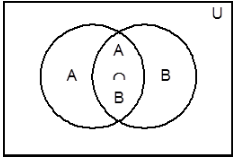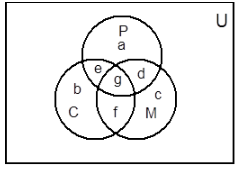Courses

# Complementary Set and Operations on Sets JEE Notes | EduRev

## JEE : Complementary Set and Operations on Sets JEE Notes | EduRev

The document Complementary Set and Operations on Sets JEE Notes | EduRev is a part of JEE category.
All you need of JEE at this link: JEE

COMPLEMENTARY SET

The complement of a set A with respect to the Universal Set U is difference of U and A. Complement of set A is denoted by  ≤ or (AC) ≤ or (A¢). Thus  is the set of all the elements of the Universal Set which do not belong to the set A.

= U – A = {x: x ∈ U and x ∉ A}

we can say that A  = U ≤ (Universal Set) and A ∩  = ϕ ≤ Void Set

Some of the useful properties/operations on sets are as follows:

• A ∪ U = U
• A ∩ ϕ = ϕ
• ϕC = U
• UC = ϕ

Algebra of Sets:

Idempotent LawFor any set A,

• A ∪ A = A
• A ∩ A = A

Identity Law: For any set A,

• A ∪ ϕ = A
• A ∩ U = A

Commutative Law: For any two sets A and B

• A ∪ B = B ∪ A
• A ∩ B = B ∩ A

Associative Law: For any three sets A, B and C

• ≤ A ∪ B) ∪ C = A ∪ ≤ B ∪ C)
• A ∩ ≤ B ∩ C) = ≤ A ∩ B) ∩ C

Distributive Law: For any three sets A, B and C

• A ∪ ≤ B ∩ C) = ≤ A ∪ B) ∩ ≤ A ∪ C)
• A ∩ ≤ B ∪ C) = ≤ A ∩ B) ∪ ≤ A ∩ C)

De Morgan’s Law: For any two sets A and B

• ≤ A ∪ B)¢ = A¢ ∩ B¢
• ≤ A ∩ B)¢ = A¢ ∪ B¢

POWER SET

The set of all subsets of a given set A is called the power set A and is denoted by P(A).

P(A) = {S: S ⊆ A}

For example, if A {1, 2, 3}, then

P(A) { ϕ,{1},{2},{3},{1,2},{1,3},{2,3},{1,2,3}}

Clearly, if A has n elements, then its power set P(A) contains exactly 2n elements.

Some More Results:

• n  (set of elements neither in A nor in B) = n  (A¢ ∩ B¢) = n (A ∪ B)¢ = n  (U) – n  (A ∪ B)
• n  (A¢ ∪ B¢) = n (A ∩ B)¢ = n ( U) – n  (A ∩ B)

• n  (A Δ B) = n [ (A – B) ∪  (B – A)] = n  [(A ∩ B¢) ∪  (A¢ ∩ B)] = n  (A) + n  (B)– 2n (A ∩ B).

The diagrams drawn to represent sets are called VennThe diagrams drawn to represent sets are called Venn diagrams or Euler -Venn diagrams. Here we represent the universal set U by points within rectangle and the subset A of the set U represented by the interior of a circle. If a set A is a subset of a set B then the circle representing A is drawn inside the circle representing B. If A and B are no equal but they have some common elements, then to represent A and B by two intersecting circles.

Illustration -: A class has 175 students. The following table shows the number of students studying one or more of the following subjects in this case

 Subjects No. of students Mathematics 100 Physics 70 Chemistry 46 Mathematics and Physics 30 Mathematics and Chemistry 28 Physics and Chemistry 23 Mathematics, Physics and Chemistry 18

How many students are enrolled in Mathematics alone, Physics alone and Chemistry alone? Are there students who have not offered any one of these subjects?

Solution: Let P, C, M denotes the sets of students studying Physics, Chemistry and Mathematics respectively.

Let a, b, c, d, e, f, g denote the number of elements ≤ students) contained in the bounded region as shown in the diagram thena + d + e + g = 70
c + d + f + g = 100
b + e + f + g = 46
d + g = 30
e + g = 23
f + g = 28
g = 18

after solving we get g = 18, f = 10, e = 5, d = 12, a = 35, b = 13 and c = 60
Therefore a + b + c + d + e + f + g = 153
So, the number of students who have not offered any of these three subjects = 175 –153 = 22
Number of students studying Mathematics only, c = 60
Number of students studying Physics only, a = 35
Number of students studying Chemistry only, b = 13.

Offer running on EduRev: Apply code STAYHOME200 to get INR 200 off on our premium plan EduRev Infinity!

,

,

,

,

,

,

,

,

,

,

,

,

,

,

,

,

,

,

,

,

,

;